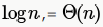Courses

# Time Complexity MCQ - 1

## 10 Questions MCQ Test RRB JE for Computer Science Engineering | Time Complexity MCQ - 1

Description
This mock test of Time Complexity MCQ - 1 for Computer Science Engineering (CSE) helps you for every Computer Science Engineering (CSE) entrance exam. This contains 10 Multiple Choice Questions for Computer Science Engineering (CSE) Time Complexity MCQ - 1 (mcq) to study with solutions a complete question bank. The solved questions answers in this Time Complexity MCQ - 1 quiz give you a good mix of easy questions and tough questions. Computer Science Engineering (CSE) students definitely take this Time Complexity MCQ - 1 exercise for a better result in the exam. You can find other Time Complexity MCQ - 1 extra questions, long questions & short questions for Computer Science Engineering (CSE) on EduRev as well by searching above.
QUESTION: 1

### The below question is based on following program:   procedure mystery (A : array [1..100] of int) int i,j,position,tmp; begin for j := 1 to 100 do position := j; for i := j to 100 do if (A[i] > A[position]) then                     position := i;                 endfor             tmp := A[j];            A[j] := A[position];             A[position] := tmp;         endfor end The number of times the test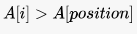is executed is:

Solution:

Ans- 5050 ( 100 +99 + 98 + ..... +1 = (100 *101)/2)

QUESTION: 2

### How many times is the comparison i > = n performed in the following program? int i=85, n=5; main() { while (i >= n) {         i=i-1;         n=n+1; } }

Solution:

It will start comparison from (85, 5), (84, 6), (83, 7) .............. (45, 45), (44, 46)
Hence total number of comparison will be (85-44) + 1 = 41 + 1 = 42

QUESTION: 3

### The complexity of merge sort algorithm is

Solution:

The worst case complexity for merge sort is O(nlogn).

QUESTION: 4

Suppose we want to arrange the  numbers stored in any array such that all negative values occur before all positive ones. Minimum number of exchanges required in the worst case is

Solution:

Answer is (d) None of these
We just require n/2 swaps in the worst case. The algorithm is as given below:
Find positive number from left side and negative number from right side and do exchange. Since, at least one of them must be less than or equal to n/2, there cannot be more than n/2 exchanges. An implementation is given below:

QUESTION: 5

If n is a power of 2, then the minimum number of multiplications needed to compute an is

Solution: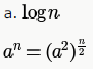One multiplication and recurrence on n/2 . So, we get the recurrence relation for the number of multiplications as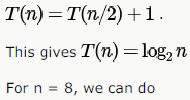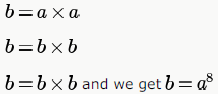QUESTION: 6

Let S be a sorted array of n integers. Let T(n) denote the time taken for the most efficient algorithm to determined if there are two elements with sum less than 1000 in S. Which of the following statement is true?

Solution:

Because array is always sorted just check the 1st two elements. option a.

QUESTION: 7

The cube root of a natural number n is defined as the largest natural number m such that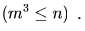The complexity of computing the cube root of n (n is represented by binary notation) is

Solution:

We can simply do a binary search in the array of natural numbers from 1...n and check if the cube of the number matches n (i.e., check if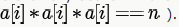This check takes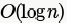time and in the worst case we need to do the searchtimes. So, in this way we can find the cube root in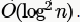So, options (A) and (B) are wrong.

Now, a number is represented in binary using logn bit. Since each bit is important in finding the cube root, any cube root finding algorithm must examine each bit at least once. This ensures that complexity of cube root finding algorithm cannot be lower than logn (It must be Ω(logn)). So, (D) is also false and (C) is the correct answer.

QUESTION: 8

Two matrices M1 and M2 are to be stored in arrays A and B respectively. Each array can be stored either in row-major or column-major order in contiguous memory locations. The time complexity of an algorithm to compute M1*M2 will be

Solution:

This is a trick question. Note that the questions asks about time complexity, not time taken by the program. for time complexity, it doesn’t matter how we store array elements, we always need to access same number of elements of M1 and M2 to multiply the matrices. It is always constant or O(1) time to do element access in arrays, the constants may differ for different schemes, but not the time complexity.

QUESTION: 9

Let A[1, …n] be an array storing a bit (1 or 0) at each location, and f(m) is a function whose time complexity is θ(m). Consider the following program fragment written in a C like language:

counter = 0;

for (i=1; i<=n; i++)

{ if a[i] == 1) counter++;

else {f (counter);

counter = 0;}

}

The complexity of this program fragment is

Solution:

The key part in the code is "counter = 0" in the else part as we can see below.

Lets take the best case. This happens when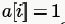for all i,and then the loop executes with time complexity θ(1) for each iteration and hence overall time complexity of θ(n) and we can say time complexity of the code fragment is Ω(n) and hence options a and b are false.

Now, consider the worst case. This happens when a[i] = 0 or when else part is executed. Here, the time complexity of each iteration will be θ(Counter) and after each else, counter is reset to 0. Let k iterations go to the else part during the worst case. Then the worst case time complexity will be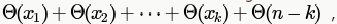where Xi is the value of the counter when,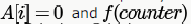is called. But due to Counter =0after each call to f(), we have,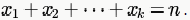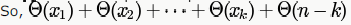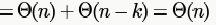Since the time complexity is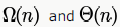we can say it is θ(n) Option (C). (Option D is false because the small o
needs the growth rate to be STRICTLY lower and not equal to or lower as the case for big 0)

IF Counter = 0was not there in else part, then time complexity would be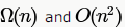as in worst case we can have equal number of 0's and 1's in array a giving time complexity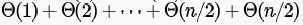would give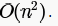QUESTION: 10

Consider the following C-program fragment in which i, j and n are integer variables.

for( i = n, j = 0; i > 0; i /= 2, j +=i );

Let val ( j ) denote the value stored in the variable j after termination of the for loop. Which one of the following is true?

Solution: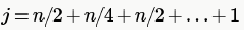number of iteration will be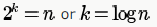this is in GP find sum till